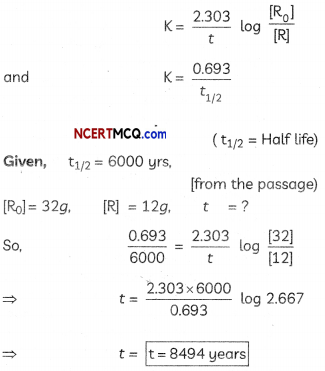Students can access the CBSE Sample Papers for Class 12 Chemistry with Solutions and marking scheme Term 2 Set 1 will help students in understanding the difficulty level of the exam.

## CBSE Sample Papers for Class 12 Chemistry Term 2 Set 1 with Solutions

Time Allowed: 2 Hours
Maximum Marks: 35

General Instructions:

• There are 12 questions in this question paper with internal choice.
• SECTION A – Q. No. 1 to 3 are very short answer questions carrying 2 marks each.
• SECTION B – Q. No. 4 to 11 are short answer questions carrying 3 marks each.
• SECTION C- Q. No. 12 is case based question carrying 5 marks.
• All questions are compulsory.
• Use of log tables and calculators is not allowed.

Section – A
(Section A-Question No 1 to 3 are very short answer questions carrying 2 marks each.)

Question 1.
Arrange the following in the increasing order of their property indicated (any 2):
(a) Benzoic acid, Phenol, Picric acid, Salicylic acid (pka values).
Picric acid < salicylic acid < benzoic acid <phenol

Explanation:

• Picric acid (2,4,6 – Trinitrophenol) – Presence of electron-withdrawing group (-NO2) increases the acidic character.
• Salicylic acid (2-Hydroxy benzoic acid) – Stability of salicylate ion due to intramolecular H-bonding.
• Benzoic acid (C6H5COOH) – More stability of benzoate ion due to resonance which is prominent on carboxylate ion.
• Phenol (C6H5OH) – Stability of phenoxide ion due to resonance.

(b) Acetaldehyde, Acetone, Methyl tert butyl ketone (reactivity towards NH2OH).
Methyl tert – butyl ketone < acetone< Acetaldehyde

Explanation:

• More the steric hinderance, difficult is the attack of nucleophile.
• Methyl tert-butyl ketone < Acetone < Acetaldehyde.
(Max. steric hinderance) (Min. steric hinderance)

(c) ethanol, ethanoic acid, benzoic acid (boiling point) (2)
ethanol

we can calculate the dilution of tube by dilution factor formula and…View the full answer.

Question 2.
Solutions of two electrolytes ‘A’ and ‘B’ are diluted. The Am of ‘B’ increases 1.5 times while that of A increases 25 times. Which of the two is a strong electrolyte? Justify your answer. Graphically show the behavior of ‘A’ and ‘B’. (2)
B is a strong electrolyte. The molar conductivity increases slowly with dilution as there is no increase in number of ions on dilution as strong electrolytes are completely dissociated.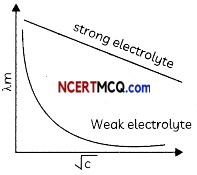Related Theory:
For strong electrolytes number of ions remains same at oil concentrations due to their complete dissociation. The ions become far apart from one another and their interionic forces decrease Hence, molar conductivity increases slowly with increase in dilution.

For weak electrolytes degree of dissociation increases with an increase in dilution, so molar conductivity increases maximum near-infinite dilution.Question 3.
Give reasons to support the answer
(a) Presence of Alpha hydrogen in aldehydes and ketones is essential for aldol condensation.
The alpha hydrogen atoms are acidic in nature due to presence of electron withdrawing carbonyl. group. These can be easily removed by a bose and the carbanion formed is resonance stabilized.

Explanation: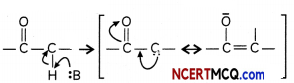(b) 3 -Hydroxy pentan-2-one shows positive Tollen’s test. (2)
Tollen’s reagent is o weak oxidizing agent not capable of breaking the C-C bond in ketones . Thus ketones cannot be oxidized using Totlen’s reagent itseLf gets reduced to Ag.

Explanation:
3-Hydroxy pentan-2-one does not give Tollen’s test

Related Theory:
Aldehydes have a proton attached to the carbonyl carbon which can be abstracted, aUowing them to be easily oxidized to form carboxylic acids. The lack of this hydrogen, makes ketones generally inert to these oxidation conditions i.e with mild oxidizing agents Like Tollen’s reagent.

Section – B
(Section B-Question No 4 to 11 are short answer questions carrying 3 marks each.)

Question 4.
Account for the following:
(a) Aniline cannot be prepared by the ammonolysis of chlorobenzene under normal conditions.
(b) N-ethylethanamine boils at 329.3K and butanamine boils at 350.8K, although both are isomeric in nature.
(c) Acylation of aniline is carried out in the presence of pyridine.
OR
Convert the following:
(a) Phenol to N-phenylethanamide.
(b) Chloroethane to methanamine.
(c) Propanenitrile to ethanol. (3)
(a) in case of chlorobenzene, the C—Cl bond is quite difficult to break as it acquires a partial double bond character due to conjugation.
So Under the normal conditions, ammonolysis of chlorobenzene does not yield aniline.

(b) Primary and secondary amines are engaged in intermolecular association due to hydrogen bonding between nitrogen of one and hydrogen of another molecule. Due to the presence of two hydrogen atoms, the intermolecular association is more in primary amines than in secondary amines as there is one hydrogen atom available for hydrogen bond formation in it

(c) During the acylation of aniline, stronger base pyridine is added. This done in order to remove the HCl so formed during the reaction and to shift the equilibrium to the right hand side.

OR

(a) Phenol into IM-pherylethanamide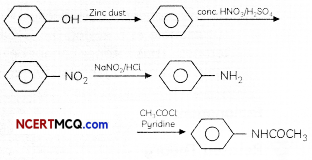(b) Chloroethane to methanamine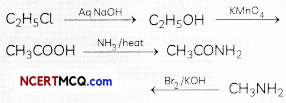(c) Propanenitrile to ethanal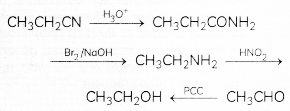Related Theory:
(a) Since aryl halides do not undergo nucleophilic substitution reactions due to resonance effect causing partial double bond character of C-X bond, so a of chiorobenzene cannot be substituted with ammonia (nucleophite).

(b) N-ethyl ethanamine CH3CH2NCH2CH3 is a secondary amine whereas butanamine (CH3CH2CH2CH2NH2) is a primary amine. Since intermolecular hydrogen bonding is more in 1° amines than those in 2° amines so boiling points of 1° amines are higher than those of2°amines.

(c) Pyridine is used to remove the side product formed in the acylation reaction i.e., HCl from the reaction mixture. Being a strong base, it acts as an acceptor for the acid by-product formed in the reaction.Question 5.
(a) [Ni(H2O)6]2+ (ag) is green in colour whereas [Ni(H2O)4 (en)]2+ (aq) is blue in colour, give reason in support of your answer.
(b) Write the formula and hybridization of the following compound: tris(ethane-1,2-diamine) cobalt(III) sulphate
OR
In a coordination entity, the electronic configuration of the central metal ion is t2g3eg1
(a) Is the coordination compound a high spin or low spin complex?
(b) Draw the crystal field splitting diagram for the above complex.
(a) The colour of coordination compound depends upon the type of ligand and d-d transition taking place.
H2O is weak field ligand, which causes small splitting, leading to the d-d transition corresponding green colour, however due to the presence of (en) which is strong field ligand , the splitting is increased . Due to the change in t2g – eg splitting the colouration of the compound changes from green to blue. :

(b) Formula of the compound is [Co(H2NCH2CH2NH2)3]2 (SO4)3
The hybridisation of the compound is: d2sp3
OR
(a) As the fourth electron enters one of the eg orbitals giving the configuration t2g3 eg1, which indicates ∆0< P hence forms high spin complex.

(b)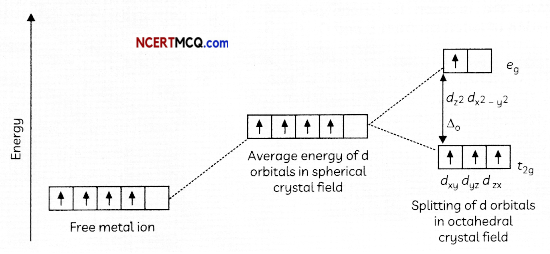Related Theory:
(a) The cause of the cotour of coordination compounds can be explained by Crystal Field Theory. The colours exhibited by transition- metal complexes are caused by

• excitation of an electron from a lower-energy d orbital to a higher-energy d orbital, which is called a d-d transition,
• The specific ligands(strong or weak) coordinated to the central metal atom.

(b) According to Valence Bond Theory (VBT), Co(en)3]2(SO4)3 forms inner orbital complex involving d2sp3 hybridization.
OR
Related Theory:
(a) According to Crystal Field Theory (CFT), given configuration t2g3 eg1 shows that splitting energy ∆o is less than pairing energy P(∆o < P) causing no pairing of electrons, so high spin complex will be formed.

Question 6.
Account for the following:
(a) Ti(IV) is more stable than Ti(II) or Ti(III).
(b) In case of transition elements, ions of the same charge in a given series show progressive decrease in radius with increasing atomic number.
(c) Zinc is a comparatively a soft metal, iron and chromium are typically hard. 3
(a) Ti is having electronic configuration [Ar] 3d2 4s2. Ti (IV) is more stable as Ti4+ acquires nearest noble gas configuration on loss of 4 e.

(b) In the case of transition elements, ions of the same charge in a given series show progressive decrease in radius with T increasing atomic number.

As the new electron enters a d orbital each time the nuclear charge increases by unity. The shielding effect of a d electron is not that effective, hence the net electrostatic attraction between the nuclear charge and the outermost electron increases and the ionic radius decreases.

(c) Iron and Chromium are having high enthalpy of atomization due to the presence of unpaired electrons, which accounts for their hardness. However, Zinc has low enthalpy of atomization as it has no unpaired electron. Hence zinc is comparatively a soft metal.

Related Theory:
(a) By losing its four electrons of 3d2 4s2, Ti4+ attains stable configuration of the nearest noble gas with the complete octet.

Caution:
(b) Students should read the question carefully. Question is asking about the trend of ionic radii of transition metal ions and not about the trend of atomic radii of transition metal atoms because both the trends are different.

Related Theory:
(c) Zn has completely filled d-orbital, hence it cannot form metallic bonds (less enthalpy of atomization),while Cr has half filled d-orbital (3d5) and Fe has partially filled d-orbital (3d6) and can form metallic bonds (high enthalpies ofatomization). Since metallic bonds make a metal hard, Cr and Fe are hard metals and Zn is a soft metal.

Question 7.
An alkene ‘A’ (Mol. formula C5H10) on ozonolysis gives a mixture of two compounds ‘B’ and ‘C’. Compound ‘B’ gives positive Fehling’s test and also forms iodoform on treatment with I2 and NaOH. Compound ‘C’ does not give Fehling’s test but forms an iodoform. Identify the compounds A, B and C. Write the reaction for ozonolysis and formation of iodoform from B and C. (3)
Compound A is an alkene, on ozonolysis, it will give carbonyl compounds. As both B and C have >C=0 group, B gives positive Fehling’s test so it is an aldehyde and it gives iodoform test so it has CH3C=0 group. This means the aldehyde is acetaldehyde. C does not give Fehling’s test, so it is a ketone. It gives a positive iodoform test so it is a methyl ketone means it has CH3C=0 group

Compound A (C5H10) on ozonlysis gives B (CH3CHO) + C (CH3COR) SO “C” is CH3COCH3• CH3CHO + 2Cu2+ + 5OH → CH3COO + Cu20 (red ppt) + 3H2O
• CH3COCH3 + 2Cu2+ + 5OH → No reaction
• CH3CHO + 3I3 + 3 NaOH → CHI3 (yellow ppt) + 3HI + HCOONa
• CH3COCH3 + 3I2 + 3 NaOH → CHI3 (yellow ppt) + 3HI + CH3COONa

A = CH3CH = C(CH3)2
B = CH3CHO
C = CH3COCH3

Related Theory:
Alkenes on ozonolysis give carbonyl compounds > C = O group.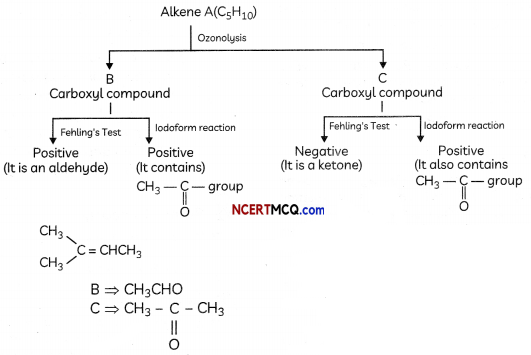Question 8.
Observe the figure given below and answer the questions that follow: semi-permeable membrane(a) Which process is represented in the figure?
electrodialysis

Explanation:
Electrodialysis is a process by which a colloidal solution is purified under the influence of electric field.

(b) What is the application of this process?
purification of colloidal solution.

Explanation:
During this process of purification, the colloidal solution containing ionic impurities is put in bag of parchment paper under electric field so that ions can pass through the parchment paper but colloidal solution does not.

(c) Can the same process occur without applying electric field? Why is the electric field applied? (3)
Yes. Dialysis is a very slow process to increase its speed electric field is applied.

Explanation:
Electrodialysis is faster process than normal dialysis under the influence of electric field.

Question 9.
What happens when reactions:
(a) N-ethylethanamine reacts with benzenesulphonyl chloride.
(b) Benzylchloride is treated with ammonia followed by the reaction with Chloromethane.
(c) Aniline reacts with chloroform in the presence of alcohoLic potassium hydroxide.
OR
(a) Write the tUPAC name for the following organic compound:(b) Complete the following: (3)(a) When N-ethylethanamine reacts with benzenesulphonyt chloride. N.N-diethylbenzenesutphonamide is formed.
(b) When benzytchloride is treated with ammonia , Benzytamine is formed which on reation with Chioromethane yields a secondary amine. N-methylbenzytamine.
(C) When aniLine reacts with chloroform in the presence of alcoholic potassium hydroxide. phenyl isocyanides or phenyt isonitrite is formed.
OR
(i) N-Ethyt-N-methytbenzenamine or N-EthyL-N-ethytaniline

(ii)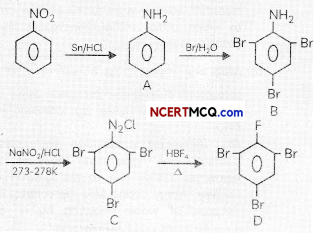Related Theory:
(a) The reaction of the benzene suiphonyl chionde (Hinsbergs reagent) with secondary amines like N-Ethylethanamine gibes a suiphonamide product (N, N-Diethylbenzenesulphonomide) that is NOT soluble ¡n alkall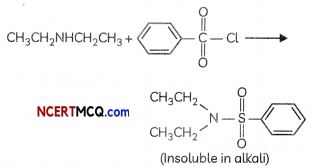(b) Benzy chloride (C6H5CH2Cl) undergoes nucleophilic substitution with ammonia (NH3) to give Benzylamine (CH5CH2NH,) which on reaction with chtorornethane (CH3Cl) undergoes alkylation to give N-methyl benzyiomine, a secondary amine.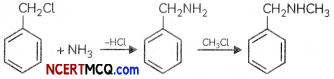(c) Carbyl amine reaction/Ho ffmonWs carbytomines test/Isocyonide test: Aliphotic or aromatic primary amines on heating with chloroform and alcoholic KOH give foul smelting alkyt isacyariides or carbytamines. This test is not given by secondary or tertiary amines.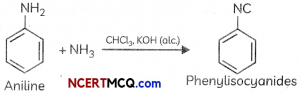Related Theory:
(a) It is a tertiary amine so alkyl groups attached to N atoms will be named first in their alphabetical order followed by the name of parent amine (with the largest olkyl group).

(b) Compound C and D given in the question are from the deleted portion of Chapter 13- AminesQuestion 10.
Represent the cell in whkh the following reaction takes place. The value of E° for the cell ¡s 1.260 V. What is the value of E?
2Al(s) + 3Cd2+(0.1M) → 3Cd(s) + 2Al3+(0.01M)
Al(s)/Cd2+(0.1M)//Al3+(0.01M)/Cd(s)
2Al(s) + 3Cd2+(0.1M) → 3Cd(s) + 2Al3+(0.01M)

Ecell = E°cell$$\frac{-0.059}{n}$$log$$\frac{\left[\mathrm{Al}^{3+}\right]^{2}}{\left[\mathrm{Cd}^{2+}\right]^{3}}$$

Ecell = 1.26 – $$\frac{0.059}{6}$$log$$\frac{(0.01)^{2}}{(0.1)^{3}}$$
= 1.26 – $$\frac{0.059}{6}$$(-1)
= 1.26 + 0.009
= 1.269 V

Caution:
Students generally forget to put powers of stoichiometric coefficients on concentration of ions in Nernst equation so see the stoichiometric coefficients carefully and try to avoid the very common mistake.

NERNST Equation:
cell = Ecell – $$\frac{0.059}{n}$$ log$$\frac{[P]^{x}}{[R]^{y}}$$

Question 11.
(a) Why are fluorides of transition metals more stable in their higher oxidation state as compared to the lower oxidation state?
(b) Which one of the following would feel attraction when placed in magnetic field: Co2+, Ag+, Ti4+, Zn2+
(c) It has been observed that first ionization energy of 5d series of transition elements are higher than that of 3d and Ad series, explain why?
OR
On the basis of the figure given below, answer the following questions:(a) Why Manganese has Lower melting point than Chromium?
(b) Why do transition metals of 3d series have Lower melting points as compared to 4d series?
(c) In the third transition series, identify and name the metal with the highest melting point. (3)
(a) The ability of fluorine to stabilize the highest Oxidation state is attributed to the higher lattice energy or high bond enthalpy.

(b) Co2+ has three unpaired electrons so it would be paramagnetic in nature, hence Co2+ ion would be attracted to magnetic field.

(c) The transition elements of 5d series have intervening 4f orbitals. There is greater effective nuclear charge acting on outer valence electrons due to the weak shielding by 4f electrons. Hence first ionisation energy of 5d series of transition elements are higher than that of 3d and 4d series.

OR

(a) Manganese is having lower melting point as compared to chromium, as it has highest number of unpaired electrons, weak interatomic metal bonding, hence no delocalisation of electrons.

(b) There is much more frequent metal – metal bonding in compounds of the heavy transition metals i.e., 4d and 5d series, which accounts for lower melting point of 3d series.

(c) Tungsten

Related Theory:
(a) Transition metal fluorides in their higher oxidation station state forms smaller cation due to which lattice enthalpy increases and so the stability increases.

(b) Magnetic moment {μ = $$\sqrt{n(n+2)}$$}of Co2+ is maximum due to the presence three unpaired electrons so attracted by external magnetic field.

(c) First ionisation stionisation enthalpies of 5d elements are higher than those of 3d or 4d elements this is due to the greater effective nuclear charge acting on outer valence electrons because of weak shielding by Af electrons causing lanthanoid contraction.

OR

Related Theory:
(a) Manganese has 5 electrons in its d-orbital with half-filled stable configuration so delocalization of the electrons. Hence these electrons are not free to participate in metallic bonding. Manganese has less heat of atomization and hence its melting point is also low.

(b) The size of 4d-series transition metal elements is Consequently, the melting point of elements larger than 3d-series transition metal elements of 3d-series is lower than the elements of (atomic size increases down the group) so force 4d-series. of attraction of nucleus towards outermost electrons becomes Less. Hence the outer most valence electron is available for strong metallic bond formation so a large amount of heat energy is required to break this type of metallic bond.Section – C
(Section C-Question No 12 is case-based question carrying 5 marks.)

Question 12.
Case Study-1
Are there nuclear reactions going on in our bodies?
There are nuclear reactions constantly occurring in our bodies, but there are very few of them compared to the chemical reactions, and they do not affect our bodies much. All of the physical processes that take place to keep a human body running are chemical processes. Nuclear reactions can lead to chemical damage, which the body may notice and try to fix.

The nuclear reaction occurring in our bodies is radioactive decay. This is the change of a less stable nucleus to a more stable nucleus. Every atom has either a stable nucleus or an unstable nucleus, depending on how big it is and on the ratio of protons to neutrons. The ratio of neutrons to protons in a stable nucleus is thus around 1:1 for small nuclei (Z < 20). Nuclei with too many neutrons, too few neutrons, or that are simply too big are unstable. They eventually transform to a stable form through radioactive decay. Wherever there are atoms with unstable nuclei (radioactive atoms), there are nuclear reactions occurring naturally. The interesting thing is that there are small amounts of radioactive atoms everywhere: in your chair, in the ground, in the food you eat, and yes, in your body.

The most common natural radioactive isotopes in humans are carbon-14 and potassium-40. Chemically, these isotopes behave exactly like stable carbon and potassium. For this reason, the body uses carbon-14 and potassium-40 just like it does normal carbon and potassium; building them into the different parts of the cells, without knowing that they are radioactive. In time, carbon-14 atoms decay to stable nitrogen atoms and potassium-40 atoms decay to stable calcium atoms.

Chemicals in the body that relied on having a carbon-14 atom or potassium-40 atom in a certain spot will suddenly have a nitrogen or calcium atom. Such a change damages the chemical. Normally, such changes are so rare, that the body can repair the damage or filter away the damaged chemicals.

The natural occurrence of carbon-14 decay in the body is the core principle behind carbon dating. As long as a person is alive and still eating, every carbon-14 atom that decays into a nitrogen atom is replaced on average with a new carbon-14 atom. But once a person dies, he stops replacing the decaying carbon-14 atoms. Slowly the carbon-14 atoms decay to nitrogen without being replaced, so that there is less and less carbon-14 in a dead body. The rate at which carbon-14 decays is constant and follows first order kinetics. It has a half-life of nearly 6000 years, so by measuring the relative amount of carbon-14 in a bone, archeologists can calculate when the person died.

All living organisms consume carbon, so carbon dating can be used to date any living organism, and any object made from a living organism. Bones, wood, leather, and write in continuation to above line paper can be accurately dated, as long as they first existed within the last 60,000 years. This is all because of the fact that nuclear reactions naturally occur in living organisms.

(source: The textbook Chemistry: The Practical Science by Paul B. Kelter, Michael D. Mosher and Andrew Scott states)
(a) Why is Carbon -14 radioactive while Carbon -12 not? (Atomic number of Carbon: 6)
(b) Researchers have uncovered the youngest known dinosaur bone, dating around 65 million years ago. How was the age of this fossil estimated?
(c) Which are the two most common radioactive decays happening in human body?
(d) Suppose an organism has 20 g of Carbon -14 at its time of death. Approximately how much Carbon -14 remains after 10,320 years? (Given antilog 0.517 = 3.289)
OR
(d) Approximately how old is a fossil with 12g of Carbon -14 If it initially possessed 32 g of Carbon -14? (Given tog 2,867 = 0.4280) (5)
(a) Ratio of neutrons to protons is 2.3:1 which is not the stable ratio of 1:1

(b) Age of fossils can be estimated by C-14 decay. All living organisms have C-14 which decays without being replaced back once the organism dies.

(c) Carbon-14 atoms decay to stable nitrogen atoms and potassium-40 atoms decay to stable calcium.

(d) t = $$\frac{2.303}{k}$$ log$$\left(\frac{\mathrm{Co}}{\mathrm{Ct}}\right)$$
Co = 20 g Ct = ?
t = 10320 gears k = $$\frac{0.693}{6000}$$ (half-Life given in passage) substituting in equation:
10320 = $$\frac{2.303}{\left(\frac{0.693}{6000}\right)}$$log$$\frac{20}{\mathrm{Ct}}$$
0.517 = Log$$\frac{20}{\mathrm{Ct}}$$ antiLog (0.517) = $$\frac{20}{\mathrm{Ct}}$$
3.289 = $$\frac{20}{\mathrm{Ct}}$$
Ct = 6.17 g.
OR
t = $$\frac{2.303}{k}$$log$$\left(\frac{\mathrm{Co}}{\mathrm{Ct}}\right)$$
Co = 32g Ct = 12
t =? k = $$\frac{0.693}{6000}$$ (half Life given in passage)
substituting in equation: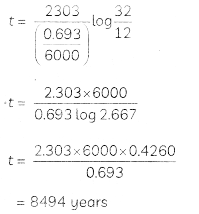(d) All nuclear reactions in radioactive decaj are of FIRST ORDER.
Formula used: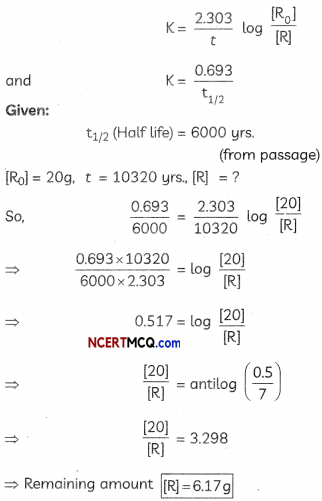Formula used: